﻿ Euler 2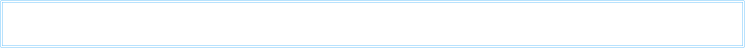Here’s a brief guide to the equation using  little more than school level maths. Euler’s symbol meanings  are as follows:-
o is  type of number called an irrational  number . Which means attempting to calculate it accurately the decimal places  would reach to infinity without  any  pattern repeat! Expressed as a fraction the same thing happens. It’s well known not only for calculating circles  etc. but also used extensively in electronics theory: roughly = 3.4142).
e is similarly another  irrational  number. It’s used heavily in so many electronics calculations. Called Euler’s number it can be used for example in calculations to describe the exponential shape of a decaying sound envelope or repeated echoes: It’s (roughly = 2.7183).
i is the square root of -1.  As multiplying either two negative or positive numbers by themselves always gives a positive result, the square root of -1 is impossible ! It can  only exist in the imagination but the imagination, as shown below, is a very powerful tool in the head of Euler. Today it  does have very important uses in maths and electronics calculations.
0 & 1 are just zero and  the first whole number or integer (not decimals or fractions). Although nothing to do with Euler’s work at the time oddly today they turn up as binary numbers, which is the basis of computer technology and digital audio.

How on earth did Euler cleverly work all this out?  First e &  o being irrational numbers, trying to calculate their true value using integers as decimal or fractions you would go on for ever slowly getting more accurate. The calculation can never fully terminate. However Euler expressed  these numbers in a very useful infinite series notational form. Doing this the numbers are are easier in the case to work with....
This is the starting point:   Euler calculates his number  e as a series of fractions that go on to infinity. The simple dots  notation  ... at the end signifies this.

Euler also noted that if you raise his number to  a power x the series to infinity becomes....

Euler then  additionally multiplies the  power x by his mysterious i = the ‘impossible’ square root of  -1, to create this....

Next  because                      then                      and  squaring   again                       and so on to infinity. similarly ...                                                  etc....
substituting  these relationships in the above equation it becomes (note the colours)....

Just rearranging the grouping so the pink and blue terms are grouped  together, it now looks like this....

Euler next cleverly shows that the values of sine and cosine  curves can  also be expressed as a series  to infinity. This is how they work out in pink and blue....

By substitution it can all simplify to this, with the strange imaginary number, importantly tying Euler’s exponential number to trigonometric functions...

Clever, but not the title  formula. However in  the special case of                      or at 180deg angle then                                        and

Substituting  this special case of  o the  formula first becomes this and then simply rearranging, the strange relationship between these numbers is finally revealed in its usual form on the right.

So not only does this formula give the strange relationship between 4 quite different types of special numbers, one of which, alarmingly,  is imaginary, there are also 4 different mathematical operations taking place  - equality, addition, multiplication, and  a number raised to a power. Each one, like the numbers themselves, is used just once. In developing the formula Euler not only does all this but along the way ties together relationships of trigonometric functions (sine and cosine) and exponential or natural logarithms and a couple of ordinary numbers: quite an achievement! Exponential, sine and cosine functions, 1’s and 0’s crop up everywhere in the electronics behind electronic music.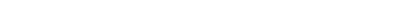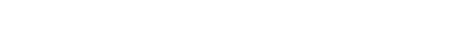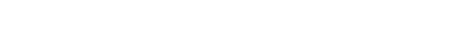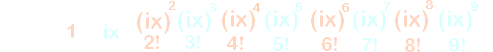Euler’s Method ...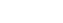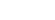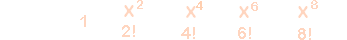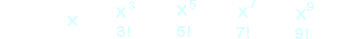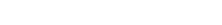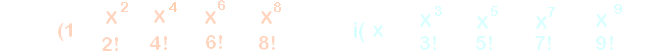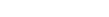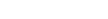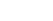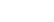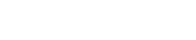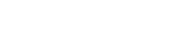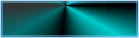| Back |  Home  |